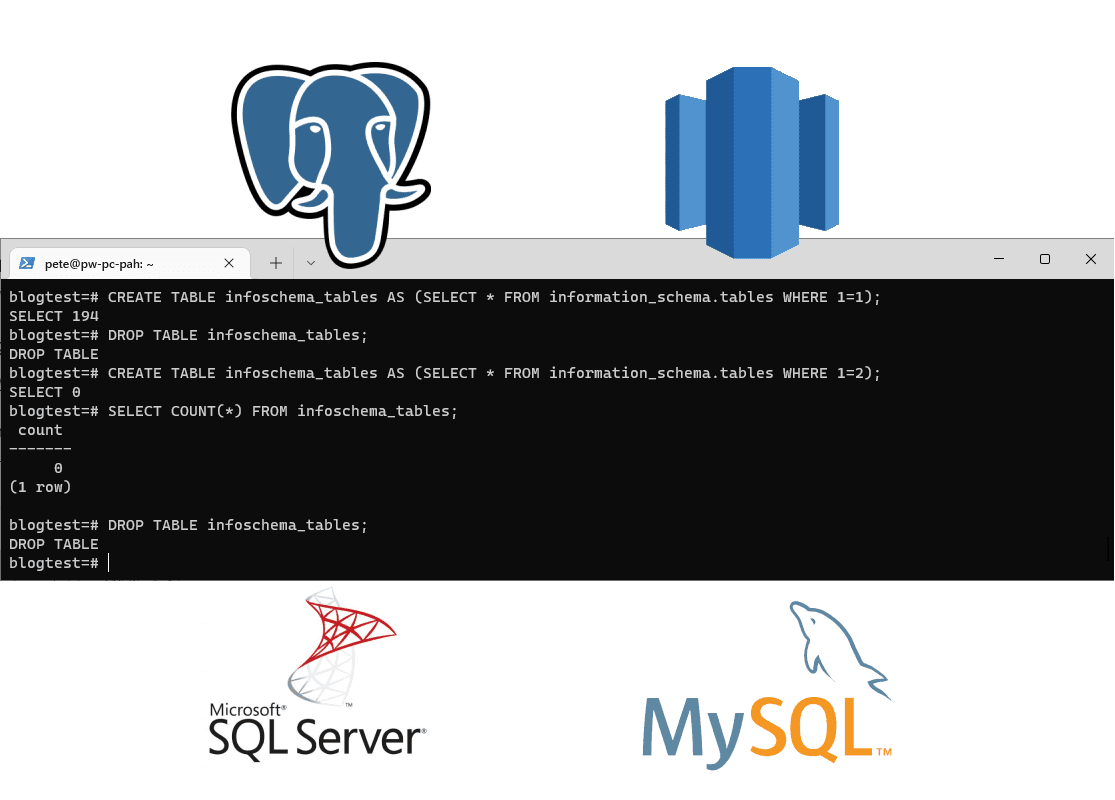# Why Use WHERE 1=2 in SQL

Some time ago I wrote a blog post on why use WHERE 1=1 in SQL. This time it’s why use WHERE 1=2, but really this can be WHERE 1=9 or anything that isn’t a 1, we just want the false statement.

WHERE 1=1 is TRUE; has no difference on execution times and can be used for ease of adding/removing comments & commas on queries that you are building.

WHERE 1=2 is FLASE; is usually used for building the structure of a table by copying it from another, without copying any of the rows. It does not copy keys & constraints.

The following example SQL should help explain this, and the SQL Syntax used below can be run on any of the SQL Systems tagged in this post (MySQL, MS SQL, Redshift, Postgres & more).

The syntax will work on other SQL systems, however, the table names used in the SQL below will not be available on all platforms. Please amend SQL table names as necessary.

```-- Create table copy from another table
CREATE TABLE infoschema_tables AS (SELECT * FROM REMOVEinformation_schema.tables WHERE 1=1);

-- Count rows of created table
-- (not required in this psql terminal example as it shows row counts)
SELECT COUNT(*) FROM infoschema_tables;

-- Drop table
DROP TABLE infoschema_tables;

-- Create table copy from another table but do not copy rows
CREATE TABLE infoschema_tables AS (SELECT * FROM REMOVEinformation_schema.tables WHERE 1=2);

-- Count rows of created table
SELECT COUNT(*) FROM infoschema_tables;

-- Clean-up drop table
DROP TABLE infoschema_tables;
```

### 1 Comment

1. […] If you wonder what would happen if we change the WHERE 1=1 to WHERE 1=2, have a look at my other blog post – Why Use WHERE 1=2 in SQL […]